# System Transmission Line

This function computes the resistive-loss of a 3-phase transmission line, and does not include the reactive components. This analysis is valid for lines up to 30 miles in length or all line voltages less than 40 kV.

## Inputs## Outputs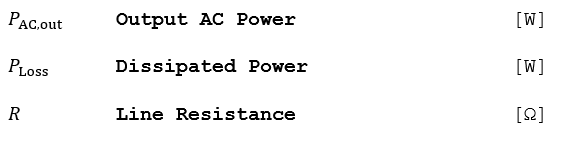## Algorithm

1.) If the input power to the transmission line is negative, set it to zero. This avoids erroneous computations at night.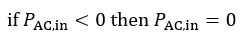2.) Find the total resistance of the line in SI units.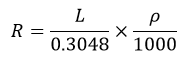3.) Find the phase current.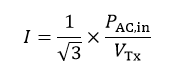4.) Find the power loss in the transmission line.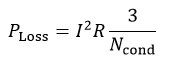5.) Find the power output. If the result is negative, power is being sourced from the grid to energize the transformers (i.e. night-time operation).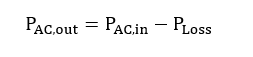Default resistance (“Resistivity”) values are populated in the PlantPredict UI based on the total system AC size, the line voltage and a Phelps-Dodge conductor sizing table.

1.) A required current rating for the line is estimated:2.) For current ratings between 145 Amps and 1200 Amps, the resistance is estimated based on a polynomial fit to the referenced Phelps-Dodge conductor sizing table.3.) Since the polynomial fit was limited to current rating values between 145 Amps and 1200 Amps, current ratings less than 145 Amps are fixed at 0.477 Ohms per 1000 feet and those greater than 1200 Amps are fixed at 0.0165 Ohms per 1000 feet.Individual data points and linear fit to Phelps-Dodge Resistance values as a function of Current Rating.

## Reference

McCann, Lawrence, ABB Electrical Transmission and Distribution Reference, Chapter 9

Phelps-Dodge, Aluminum Conductors, Aluminum-Clad Steel Reinforced. www.pdic.com. PDIC01048. 4/29/05.